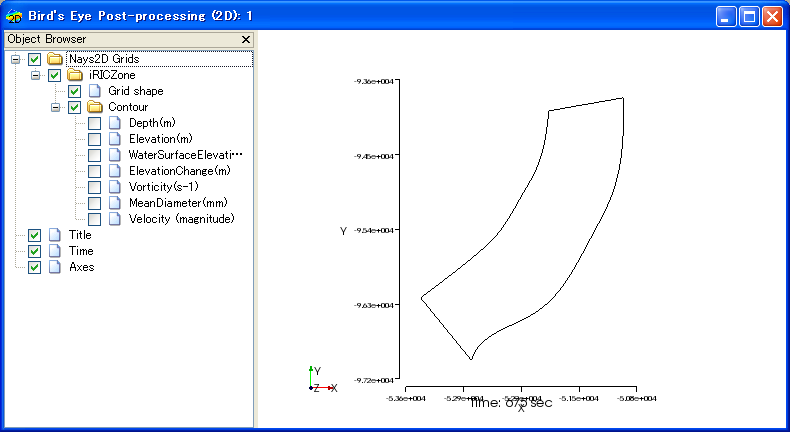iRIC functions¶

iRIC functions can be categorized into the seven groups below:

• Editing geographic data
• Creating grids
• Editing grids
• Setting calculation conditions
• Lunching a solver
• Visualizing the calculation results
• Making a graph

The abstract of each function groups are explained in the following sections.

Editing geographic data¶

Geographic data handles coordinates of data and the attributes at that coordinates, such as elevation, vegetation type, vegetation density, land use. You can import and edit geographic data with iRIC.

Geographic data are used to determine the attributes at each node or cell by interpolation. You can use cross-section data also for creating a grid.

The attributes that you need to input differ by the solver you use.

iRIC can import and edit four types of geographic data:

• Point cloud data
• Cross-section data
• Raster data
• Time series raster data
• Polygons
• Lines
• Points

Figure 18, Figure 19, Figure 20, Figure 21, Figure 22 and Figure 23 show example of [Point Cloud Data], [Cross-Section Data], [Raster Data], [Polygons], [Lines] and [Points] respectively.

Refer to [Geographic Data] for detail.

Creating a grid¶

You can create the grid that the solver uses. A grid can be created in two steps:

1. Determine the grid shape (coordinates of each node).
2. Determine the node/cell attributes by interpolating geographic data.

In step 1., you select one of the algorithms that can produce the grid that the solver requires, and then, you create a grid by specifying grid creating condition.

Step 2. is automatically done. iRIC does this step automatically by recognizing the type of geographic data, and selecting the appropriate algorithm for interpolation for that type.

iRIC can create grids of the following types:

• Two-dimensional structured grid
• Two-dimensional unstructured grid
• One-dimensional structured grid (Each node holds sectional data.)

Refer to Grid creating functions for details.

Editing the grid¶

You can edit the grid. You can do the following operations:

• Editing the grid shape (the coordinates of each node)
• Editing the attributes of each node or cell

Refer to Editing the grid for the details.

You can load measured data from text files, to use it as background data for creating data, or to compare with calculation results. You can do the following operations:

• Importing measured data from text files
• Editing display settings of measured data

Refer to [Measured Data] (M) for the details.

Setting the calculation conditions¶

You can set the calculation conditions. The calculation conditions differ by the solver selected.

Refer to [Calculation Conditions] for the details.

Launching the solver¶

You can launch the solver and monitors the simulation status using [Solver Console]. You can stop calculations when you want to. Figure 24 shows an example of the [Solver Console] that displays solver outputs.

Refer to [Simulation] (S) for details.

Post-processing¶

You can visualize the calculation results that the solver output. You can use [2D Post-processing Window] (Figure 25), [Bird’s-Eye 2D Post-processing Window] (Figure 26), and [3D Post-processing Window] (Figure 27) for that purpose.

Refer to Visualization functions for details.Figure 26 [Bird’s-Eye 2D Post-processing Window]

Making a graph¶

You can display graphs with the calculation results that the solver output, using [Graph Window] (Figure 28) and [Scattered Chart Window] (Figure 29).

Refer to Making a graph for details.